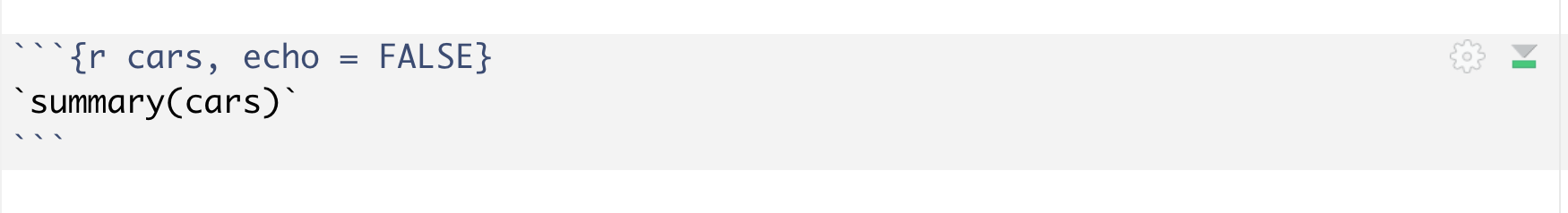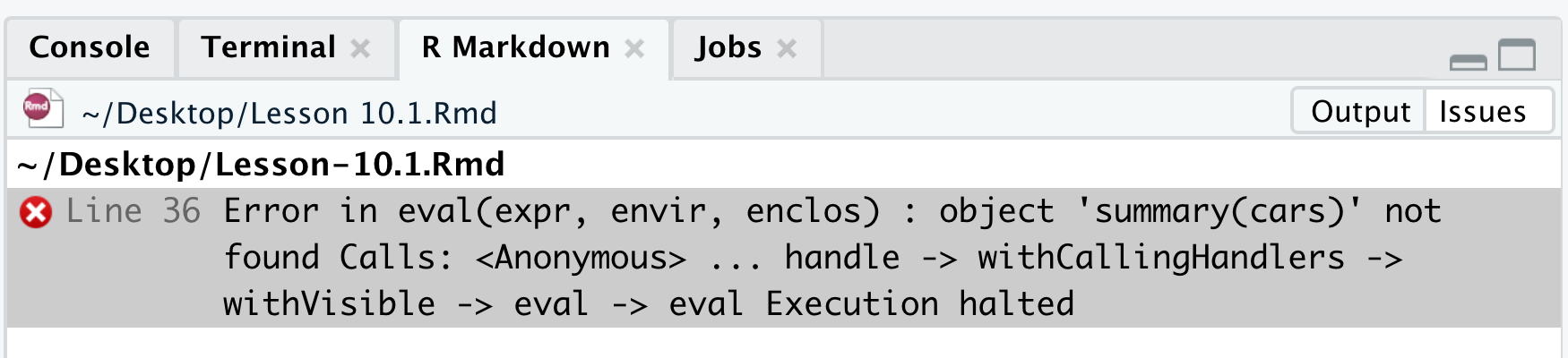## 12.2 Text Formatting in R Markdown

Having learned the YAML Header and R Code Chunks in Section 12.1, we will introduce how to format text in R Markdown document, which is crucial in writing a report.

In particular, R Markdown follows the syntax of Pandoc’s Markdown, a set of markup annotations for plain text files. Pandoc (https://pandoc.org/) is a universal document converter that converts documents from one markup format into another. Here, text in the .Rmd file is called the marked up text, and text in the output document is called the formatted text. When you knit the .Rmd file, Pandoc transforms the marked up text into formatted text in the specified file format.

In this section, we will introduce how to get several kinds of formatted text in the output document by compiling the .Rmd file.

### 12.2.1 Special text formats

Firstly, let’s discuss how to generate a couple of special formatted text.

You may use different numbers of “#” before the marked up text to generate different header levels in the output document. (with more “#”s indicating lower levels and smaller fonts). For example, you can try to the following lines in the .Rmd file and see what happens in the output document,

# Header1
###### Header6

b. change the text into italic type

To make the formatted text into italic type, you can simply use a pair of * around the text with no space. For example *italic* in the .Rmd file generates italic in the output document.

c. change the text into bold type

To make the formatted text into bold type, you can simply use a pair of ** around the marked up text with no space. For example **bold** in the .Rmd file generates bold in the output document.

d. create inline code

emphasize texts by marking them as inline codes, not recommend Sometimes you may want to mark text or code as inline code. To do that, you need to use a pair of  around the text or code. We have seen many inline codes so far. For example, *italic* is an inline code. Another common usage is when you want to include code along with other text outside code chunks. For example 1 + 1 in the markup text generates 1 + 1 in the formatted text. Here, we only display the code without running it. If you want to show the output instead of code, you can use r . For example, r 1 + 1 will generate 2 in the formatted text.

Note that if you add a pair of  around code inside the the code chunk, you will get an error message when you knit the file.Figure 12.13: Error message (I)Figure 12.14: Error message (II)

To insert a hyperlink in the output document, you can use the format [link](address) in the .Rmd file. For example, [r02pro](https://r02pro.github.io/) in the .Rmd file will generate the text r02pro with hyperlink to this book in the output document.

f. write latex equations

When writing documents that contain math symbols or equations, you can use latex equations directly in an R Markdown file, just like writing a .tex file. For example, you can use $\sum_{i=1}^{10} i = 55$ in the .Rmd file to get $$\sum_{i=1}^{10} i= 55$$ in the output document. You can also use a pair of $$ to write in display math mode. Say $$\sum_{i=1}^{10} i = 55$$ in the .Rmd file will generate $\sum_{i=1}^{10} i = 55$ in the output document. Lastly, please see the an example which has all components introduced in this part. You can copy the following lines and paste them on an R Markdown document, then click the knit button to see what will you get in the ouput document. # H1 *This is an example.* ## H2 I **learned** to use a <- rnorm(1) to generate normal distributed random variable in [r02pro](https://r02pro.github.io/). The result of 1 + 1 is 2. ### H3 I learned how to sum up numbers when I was young. For example,$$\sum_{i=1}^{10} i = 55

### 12.2.2 Lists in R Markdown

Secondly, we will introduce how to generate lists in the output document. There are two kinds of lists, unordered lists and ordered lists.

Generally, To create an unordered list, you can use the symbol *, +, or - at the beginning of the line with a space between the symbol and the text. To create higher order lists, you just need to add extra indent at the beginning of the line. Different layers have different indents and you need to align the items of the same order. Normally, there are at most three layers in a list. Please feel free to try the following example and edit it to your need.

* Item 1
* Item 2
+ Item 2a
- Item 2a.a
- Item 2a.b
+ Item 2b
* Item 3
+ Item 3a

You will see the following output in the formatted text.

• Item 1
• Item 2
• Item 2a
• Item 2a.a
• Item 2a.b
• Item 2b
• Item 3
• Item 3a

To create an ordered list, you can use numbers followed by the contents with a space in between. Similar to unordered list, you can add extra layers as needed. Please see the following example with some Spanish numbers.

1. uno
2. dos
2.1. dos.uno
2.2. dos.dos
3. tres
3.1. tres.uno
3.2. tres.dos  

You will see the following output.

1. uno
2. dos
2.1. dos.uno
2.2. dos.dos
3. tres
3.1. tres.uno
3.2. tres.dos

Of course, you can also mix ordered and unordered lists. Feel free to try the following example in your .Rmd file.

1. Item 1
2. Item 2
+ Item 2a
- Item 2a.a
- Item 2a.b
+ Item 2b
3. Item 3
+  Item 3a

### 12.2.3 Tables in R Markdown

In addition to lists, you can easily create tables using R Markdown via the kable() function in the knitr package. You need to install the knitr package if you haven’t done so.

You can study the following example and adapt it to your needs.

{r echo = FALSE, results = TRUE}
Code  <- c("dnorm(x, mean, sd)","pnorm(q, mean, sd)","qnorm(p, mean, sd)","rnorm(n, mean, sd)")
Name <- c("probability density function", "cumulative distribution function", "quantile function", "random number generator")
d <- data.frame(Code, Name)
knitr::kable(d)

Code Name
dnorm(x, mean, sd) probability density function
pnorm(q, mean, sd) cumulative distribution function
qnorm(p, mean, sd) quantile function
rnorm(n, mean, sd) random number generator

### 12.2.4 Insert citations and manage bibliographies in R Markdown

R Markdown provides us the capability to add bibliography and manage citations with ease, similar to writing a LaTex document. To add citations, you first need to add the bibliography filed in the YAML head. If your references are in the file “references.bib,” you can set the bibliography file as follows.

---
output: html_document
bibliography: references.bib
---

The .bib file is a plain-text file that consists of bibliography entries like the following:

@book{r02pro,
title = {R Programming: From Zero to Pro},
author = {r02pro-ers},
organization = {New York University},
}
Then, each bibtex items can be cited directly within the documentation using the syntax @key, where key is the citation key in the first line of the entry, e.g., @r02pro will show as in the document. To put citations in parentheses, use [@key]. To cite more than one items, separate the keys by semicolons, e.g., [@key-a; @key-b; @key-c]. To suppress the author name, you can add a minus sign before @, e.g., [-@r02pro] will generate (2021).